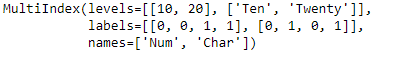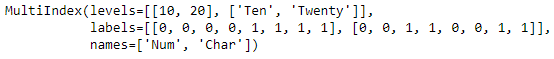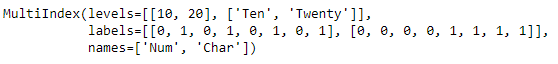Skip to content
Related Articles
Python | Pandas MultiIndex.to_hierarchical()
• Last Updated : 24 Dec, 2018

Python is a great language for doing data analysis, primarily because of the fantastic ecosystem of data-centric python packages. Pandas is one of those packages and makes importing and analyzing data much easier.

Pandas` MultiIndex.to_hierarchical()` function return a MultiIndex reshaped to conform to the shapes given by n_repeat and n_shuffle. It is useful to replicate and rearrange a MultiIndex for combination with another Index with n_repeat items.

Syntax: MultiIndex.to_hierarchical(n_repeat, n_shuffle=1)

Parameters :
n_repeat : Number of times to repeat the labels on self
n_shuffle : Controls the reordering of the labels. If the result is going to be an inner level in a MultiIndex, n_shuffle will need to be greater than one. The size of each label must divisible by n_shuffle

Returns : MultiIndex

Example #1: Use `MultiIndex.to_hierarchical()` function to repeat the labels in the MultiIndex.

 `# importing pandas as pd``import` `pandas as pd`` ` `# Create the MultiIndex``midx ``=` `pd.MultiIndex.from_tuples([(``10``, ``'Ten'``), (``10``, ``'Twenty'``),``                                  ``(``20``, ``'Ten'``), (``20``, ``'Twenty'``)], ``                                       ``names ``=``[``'Num'``, ``'Char'``])`` ` `# Print the MultiIndex``print``(midx)`

Output :Now let’s repeat the labels of the MultiIndex 2 times.

 `# repeat the labels in the MultiIndex 2 times.``midx.to_hierarchical(n_repeat ``=` `2``)`

Output :As we can see in the output, the labels in the returned MultiIndex is repeated 2 times.

Example #2: Use `MultiIndex.to_hierarchical()` function to repeat as well as reshuffle the labels in the MultiIndex.

 `# importing pandas as pd``import` `pandas as pd`` ` `# Create the MultiIndex``midx ``=` `pd.MultiIndex.from_tuples([(``10``, ``'Ten'``), (``10``, ``'Twenty'``), ``                                 ``(``20``, ``'Ten'``), (``20``, ``'Twenty'``)],``                                       ``names ``=``[``'Num'``, ``'Char'``])`` ` `# Print the MultiIndex``print``(midx)`

Output :Now let’s repeat and reshuffle the labels of the MultiIndex 2 times.

 `# resetting the labels the MultiIndex``midx.to_hierarchical(n_repeat ``=` `2``, n_shuffle ``=` `2``)`

Output :As we can see in the output, the labels are repeated as well as reshuffled twice in the returned MultiIndex.

Attention geek! Strengthen your foundations with the Python Programming Foundation Course and learn the basics.

To begin with, your interview preparations Enhance your Data Structures concepts with the Python DS Course. And to begin with your Machine Learning Journey, join the Machine Learning – Basic Level Course

My Personal Notes arrow_drop_up